This cache has been archived.

rosebud55112: Clearing out this space for someone else as described in my earlier post. Thanks to all the finders!

More
<

Filius Bonacci

A cache by rosebud55112 Message this owner
Hidden : 11/18/2009
Difficulty: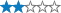Terrain: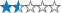Size: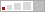(micro)

Watch

How Geocaching WorksPlease note Use of geocaching.com services is subject to the terms and conditions in our disclaimer.

Geocache Description:

NOT AT POSTED COORDINATES Micro container with logbook only. Bring your own writing utensil.

The Fibonacci series is perhaps one of the most well-known sequences of integers in mathematics. The sequence, which begins (1, 1, 2, 3, 5, 8, 13, 21, 34, 55, 89, 144……) was written about by mathematician Leonardo of Pisa (Fibonacci -Filius Bonacci, or son of Bonacci) in his book Liber Abaci in 1202, although the sequence was already well-known in Indian mathematics. The sequence may look like a random set of increasing integers, but upon closer inspection a few interesting items may be noticed:

1.) Every third number in the sequence is even, while all others are odd.
2.) Each number is the sum of the two prior numbers.
3.) The square of any number in the sequence is either one more or one less than the product of its two neighbors.

One thing not immediately obvious is that the ratio between consecutive numbers in the sequence approaches a number mathematicians call phi. Phi is approximately equal to 1.618033989…. and is exactly equal to ½ x (1+sqrt(5)). The reason that this ratio is approached is beyond the level needed for searching for this cache, but further information can be found on wikipedia.

The Fibonacci series and phi appear in the natural world underlying such things as population and plant growth, as well as in spirals and helices. Note also that phi is approximately equal to the typical scaling factor used on many GPSr maps: 20ft, 30ft, 50ft, 80ft, 120ft, 200ft, 300ft, 500ft, .2mi, .3mi, etc.

The entire Fibonacci series can be described with three equations:

1.) F(1) = 1
2.) F(2) = 1
3.) F(n+1) = F(n) + F (n-1)

These three formulas together allow any Fibonacci series number to be calculated, although it does require that all prior numbers be calculated. Sometimes ‘F(0)=0’ is included in the definition of the series, in which case ‘F(2) = 1’ becomes extraneous.

That describes “The” Fibonacci series. However, there are an infinite number of series which can be defined similarly, such as:

1.) B(1) = 7
2.) B(2) = 2
3.) B(n+1) = B(n) + B(n-1)

which leads to the series 7, 2, 9, 11, 20, 31, 51, 82, 133, 215…………

Note that for the B-series, or any series defined with the third constraint, the ratio between consecutive numbers still approaches phi. Note also that in the B-series, B(1) is greater than B(2). Series can also be created where some or all of the members are negative, such as:

1.) N(1) = 1516
2.) N(2) = 789
3.) N(n+1) = N(n) + N(n-1)

Or

1.) W(1) = -1314
2.) W(2) = 3040
3.) W(n+1) = W(n) + W(n-1)

Cache is located at N(19), W(20)

Find...

54 Logged Visits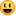43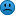3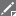3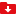1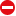1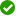1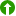1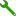1

**Warning! Spoilers may be included in the descriptions or links.Loading Cache Logs...

Current Time:
Last Updated:
Rendered From:Unknown
Coordinates are in the WGS84 datum